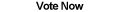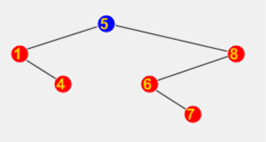Home Articles Components Code Snippets LinksSubmit a Comment

# C# AVL Tree## AVL Tree

An AVL Tree in C# is a specialized Binary Search Tree that improves the worst-case running time by self-balancing. Since an AVL Tree is a specialized Binary Tree, the AVL Tree class is built upon the C# Binary Tree class.

An AVL Tree has the advantage that operations have the same running time across different scenarios. A regular Binary Tree on the other hand has a good expected running time, but has a worst-case running time of that of a LinkedList.

The AVL Tree achieves its stable running time by self-balancing.

## Balanced AVL Tree

The balance of any node on a C# AVL Tree (or any kind of binary tree) is given by:

height of the right subtree - height of the left subtree

By subtracting the difference in heights of the children nodes, the balance of a given node can be calculated. As expected, a low number means that the difference between heights is low, making the AVL Tree balanced. Because an AVL Tree is constantly rebalancing itself, the balance of any given node will always be one of the following values: 0, -1, 1, -2, 2

In all cases, a balance of 2 or -2 means the node is unbalanced and the tree has to be rebalanced.

## Node Rotations

An AVL Tree is rebalanced by rotating segments of nodes. An AVL Tree rotation is done by shifting the parent-child relations of a node and it's left or right subtree, depending on whether the rotation is done left or right.

When an AVL Tree is unbalanced, it will always be due to one of four cases. Each case is handled with different rotations to rebalance the tree:

Right-Right: The right subtree outweighs because of a right leaf node. A single left rotation balances the tree.

Right-Left: The right subtree outweighs because of a left leaf node. To balance the right child node is rotated right, then the parent node is rotated left.

Left-Left: The left subtree outweights because of a left leaf node. A single right rotation balances the tree.

Left-Right: Similarly to the Right-Left, except a left rotation is done first followed by a right rotation.

Each of the four cases above can be determined by the balance numbers alone, since a positive balance number means the right subtree outweighs the left and a negative number means the opposite.

Also note that none of the rotations will violate the basic principles of an AVL Tree, elements in the left subtree will still be smaller than elements in the right subtree.

## Balancing an AVL Tree

There is only two times when balancing the AVL Tree is necessary: insertion and removal.

An insertion requires that the balance be checked because inserting to one side of the tree might make a subtree "heavier" to its counterpart. Once a node is inserted, the balance of every parent going up the tree until the root has to be double checked.

Similarly a removal can cause a subtree to become "lighter" an unbalance the tree. The same method is applied to rebalance.

A special case when rechecking balances is when the balance is 1 or -1. A balance of 1 or -1 indicates that the height of the tree has not changed, at which case there is no need to keep checking the balance of any more nodes.

## Other Tree Operations

Since the C# AVL Tree inherits the original Binary Tree, all normal tree operations work as normal (traversals, etc.)...

Back to C# Article List

Other C# Articles